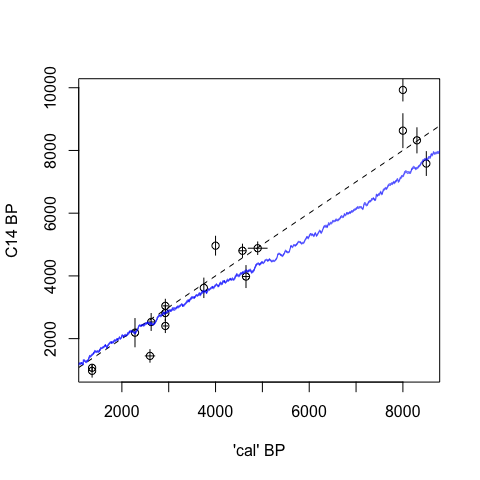# 2. plots

We can also plot a calibration curve:

draw.ccurve()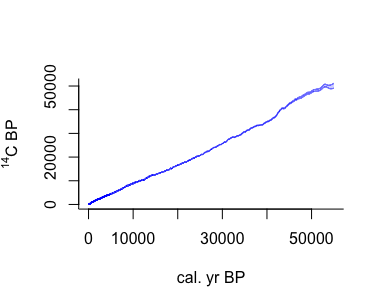Or compare two calibration curves:

draw.ccurve(1600, 2020, BCAD=TRUE, cc2='nh1', add.yaxis=TRUE)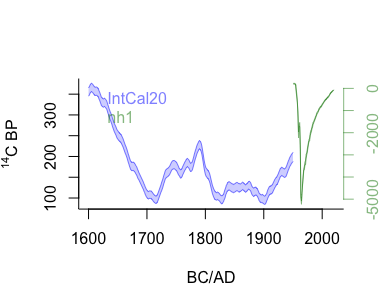Or zoom in to between AD 1600 and 2000 (using the BCAD scale):

draw.ccurve(1600, 1950, BCAD=TRUE)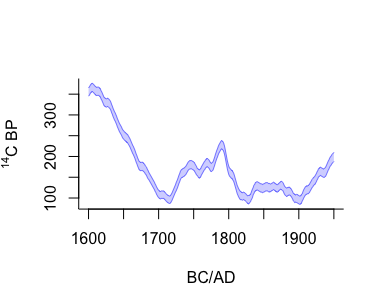Interesting things happened after 1950, as can be seen by adding a postbomb curve:

draw.ccurve(1600, 2020, BCAD=TRUE, cc2='nh1')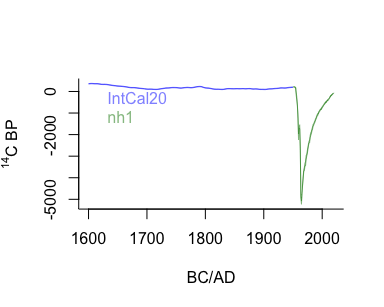The postbomb curve dwarfs the IntCal20 curve, so we could also plot both on separate vertical axes:

draw.ccurve(1600, 2020, BCAD=TRUE, cc2='nh1', add.yaxis=TRUE)We can also visualise the data underlying parts of the IntCal calibration curve, for example from 500 to 0 cal BP:

intcal.data(0, 500)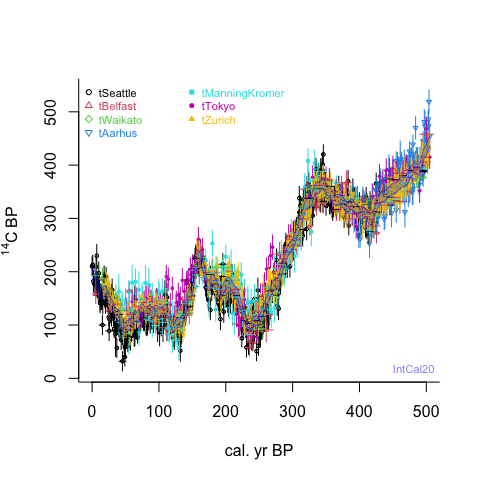Want to plot only some specific datasets over a period of time?

dat <- intcal.data(20e3, 25e3)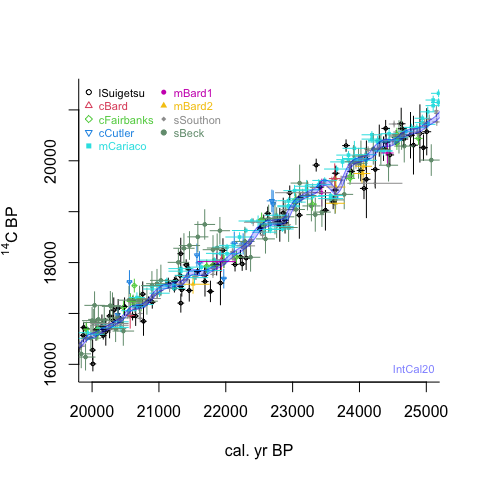unique(dat\$set)
##  109 110 111 114 120 121 122 124 126
dat <- intcal.data(20e3, 25e3, select.sets=c(109, 120), data.cols=c(1,2))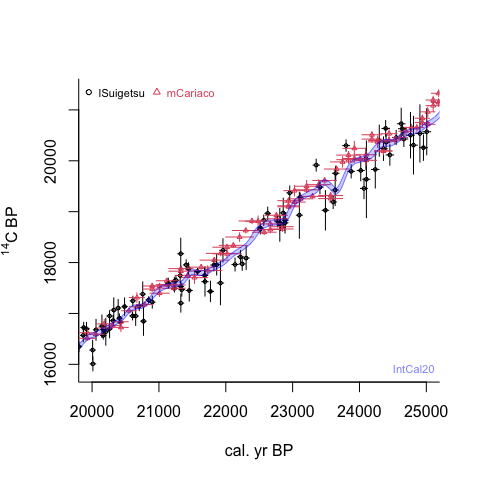Legacy calibration data such as Arnold & Libby 1951 (Science 113, p. 111-120) can be compared with the latest calibration curves. Only included here are those radiocarbon dates of Arnold and Libby which come with independent (historical, archaeological or dendrochronological) age estimates. Note that Arnold and Libby did not define their ‘calendar years’ explicitly, so the ages have to be taken with a degree of caution.

libby <- read.table(file.path(system.file(package = 'rintcal'), "extdata/Arnold_Libby_1951.txt"), header=T, sep=",")
plot(libby[,2], libby[,4], xlab="'cal' BP", ylab="C14 BP") # plot the radiocarbon dates and their known calendar ages
segments(libby[,2]-libby[,3], libby[,4], libby[,2]+libby[,3], libby[,4]) # calendar error bars (not all are quantified)
segments(libby[,2], libby[,4]-libby[,5], libby[,2], libby[,4]+libby[,5]) # radiocarbon error bars
abline(0, 1, lty=2)
draw.ccurve(0, 10e3, add=TRUE) # add the most recent calibration curve